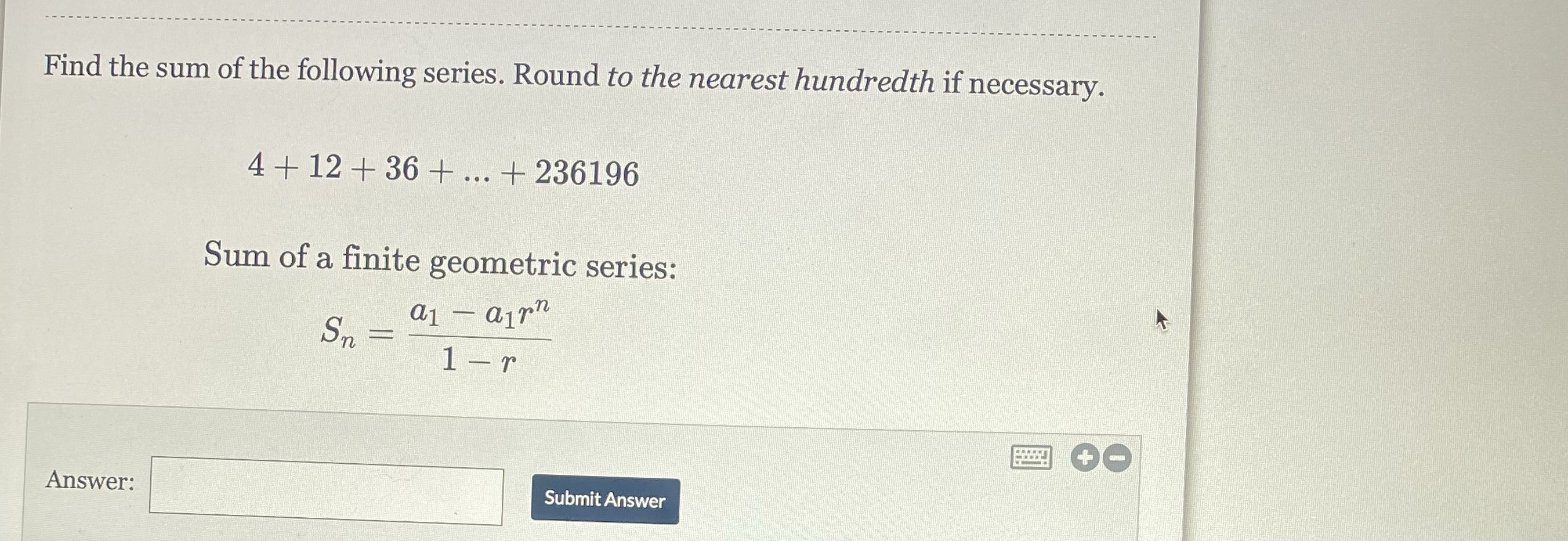Still have math questions?

Algebra
Question

Find the sum of the following series, Round to the nearest hundredth if necessary.

4+12+36+...+234196

Sum of a finite geometric series:

$$S_n=\frac{a_1-a_1r^n}{1-r}$$